Courses

# Design Of Joint - MCQ Test 1

## 20 Questions MCQ Test Mock Test Series - Mechanical Engineering (ME) for GATE 2020 | Design Of Joint - MCQ Test 1

Description
This mock test of Design Of Joint - MCQ Test 1 for Mechanical Engineering helps you for every Mechanical Engineering entrance exam. This contains 20 Multiple Choice Questions for Mechanical Engineering Design Of Joint - MCQ Test 1 (mcq) to study with solutions a complete question bank. The solved questions answers in this Design Of Joint - MCQ Test 1 quiz give you a good mix of easy questions and tough questions. Mechanical Engineering students definitely take this Design Of Joint - MCQ Test 1 exercise for a better result in the exam. You can find other Design Of Joint - MCQ Test 1 extra questions, long questions & short questions for Mechanical Engineering on EduRev as well by searching above.
QUESTION: 1

### Square key of side "d/4" each and length l is used to transmit torque "T" from the shaft of diameter "d" to the hub of a pulley. Assuming the length of the key to be equal to the thickness of the pulley, the average shear stress developed in the key is given by

Solution:

If a square key of sides d/4 is used then. In that case, for shear failure we
have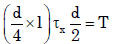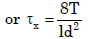[Where τx is the yield stress in shear and l is the key length.]

QUESTION: 2

### A 60 mm long and 6 mm thick fillet weld carries a steady load of 15 kNalong the weld. The shear strength of the weld material is equal to 200MPa. The factor of safety is

Solution: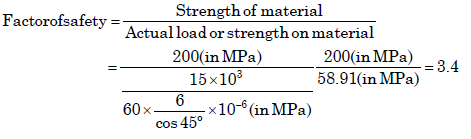QUESTION: 3

### Assertion (A): A cotter joint is used to rigidly connect two coaxial rods carrying tensile load. Reason (R): Taper in the cotter is provided to facilitate its removal when it fails due to shear.

Solution:

A cotter is a flat wedge shaped piece of rectangular cross-section and its width is tapered (either on one side or both sides) from one end to another for an easy adjustment. The taper varies from 1 in 48 to 1 in 24 and it may be increased up to 1 in 8, if a locking device is provided. The locking device may be a taper pin or a set screw used on the lower end of the cotter. The cotter is usually made of mild steel or wrought iron. A cotter joint is a temporary fastening and is used to connect rigidly two co-axial rods or bars which are subjected to axial tensile or compressive forces.

QUESTION: 4

Match List I with List II and select the correct answer using the code given below the Lists: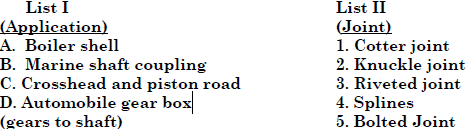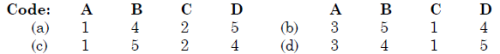Solution:
QUESTION: 5

In a gib and cotter joint, the gib and cotter are subjected to

Solution: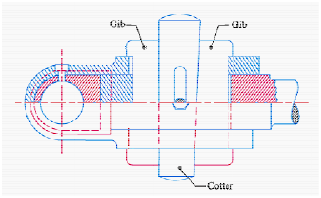QUESTION: 6

In a cotter joint, the width of the cotter at the centre is 50 mm and its thickness is 12 mm. The load acting on the cotter is 60 kN. What is the shearing stress developed in the cotter?

Solution:

It is a case of double shear.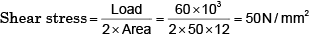QUESTION: 7

Match List-l (Machine element) with List-II (Cause of failure) and select the correct answer using the codes given below the lists: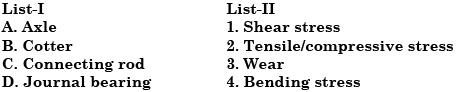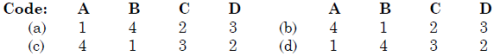Solution:

• In machinery, the general term “shaft” refers to a member, usually of circular cross-section, which supports gears, sprockets, wheels, rotors, etc., and which is subjected to torsion and to transverse or axial loads acting singly or in combination.

• An “axle” is a non-rotating member that supports wheels, pulleys, and carries no torque.

• A “spindle” is a short shaft. Terms such as line-shaft, head-shaft, stub shaft, transmission shaft, countershaft, and flexible shaft are names associated with special usage.

QUESTION: 8

A cotter joint is used when no relative motion is permitted between the rods joined by the cotter. It is capable of transmitting

Solution:
QUESTION: 9

Assertion (A): When the coupler of a turn buckle is turned in one direction both the connecting rods either move closer or move away from each other depending upon the direction of rotation of the coupler.

Reason (R): A turn buckle is used to connect two round rods subjected to tensile loading and requiring subsequent adjustment for tightening or loosening.

Solution: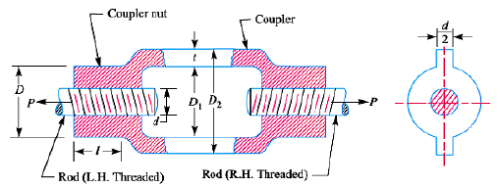QUESTION: 10

A spur gear transmitting power is connected to the shaft with a key of rectangular section. The type (s) of stresses developed in the key is fare.

Solution:

Key develops both shear and bearing stresses.

QUESTION: 11

Which key is preferred for the condition where a large amount of impact torque is to be transmitted in both direction of rotation?

Solution:
QUESTION: 12

A square key of side d/4 is to be fitted on a shaft of diameter d and in the hub of a pulley. If the material of the key and shaft is same and the two are to be equally strong in shear, what is the length of the key?

Solution:
QUESTION: 13

A pulley is connected to a power transmission shaft of diameter d by means of a rectangular sunk key of width wand length ‘l’. The width of the key is taken as d/4. For full power transmission, the shearing strength of the key is equal to the torsional shearing strength of the shaft. The ratio of the length of the key to the diameter of the shaft (l/d) is

Solution: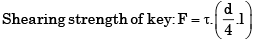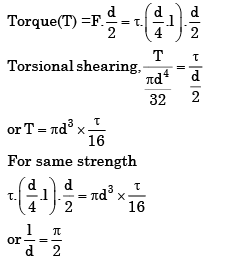QUESTION: 14

The key shown in the above figure is a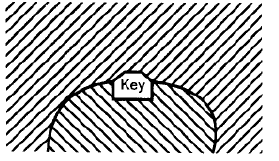Solution:
QUESTION: 15

The shearing area of a key of length 'L', breadth 'b' and depth 'h' is equal to

Solution:
QUESTION: 16

In a fillet welded joint, the weakest area of the weld is

Solution:
QUESTION: 17

Two metal plates of thickness ’t’ and width 'w' are joined by a fillet weld of 45° as shown in given figure.When subjected to a pulling force 'F', the stress induced in the weld will be

Solution:
QUESTION: 18

In the welded joint shown in the given figure, if the weld at B has thicker fillets than that at A, then the load carrying capacity P, of the joint will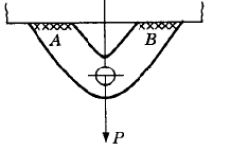Solution:
QUESTION: 19

The following two figures show welded joints (x x x x x indicates welds), for the same load and same dimensions of plate and weld.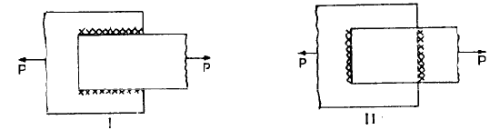The joint shown in

Solution:

Figure II is better because the weld is in tension and safe stress of weld in tension is greater than shear.

QUESTION: 20

Two plates are joined together by means of single transverse and double parallel fillet welds as shown in figure given above. If the size of fillet is 5 mm and allowable shear load per mm is 300 N, what is the approximate length of each parallel fillet?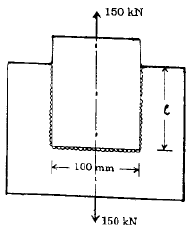Solution:

300 × (100+ 2l) = 15000 or l = 200